# Calculating Standard Deviation

## Formula for calculation in Stocks and Shares

The calculation of the standard deviation is reasonably complex, but don't worry - good stock analysis programs will be able to do the necessary calculations for you. However, I feel it's alway nice to know exactly how to calculate these things as it helps to understand the inner workings and background of how the figures are calculated.

The mathematical formula is as follows: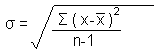We'll go through this step by step so don't be put off if it looks horrendous.

Running through the meaning of the signs, the lower case sigma, σ, indicates Standard Deviation. The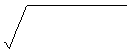sign means square root. Upper case sigma, denoted by &Sigma, means "the sum of". x is each number in the data sample, and x-bar, denoted by the sign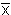is the average (also known as the "mean") of the data set. n is simply the number of values in the data sample

As with most stock analysis tools, a period of time needs to be decided upon for the sample of data. For an example, we'll use 10 days worth of data. This will give the value of n as 10. We'll be calculating the 10 day Standard Deviation for the UK company, Royal Bank of Scotland Group PLC, at the close of trade on 14 December 2004. The prices for the 10 trading days up to and including the 14 December 2004 were:

 Date in December 2004 1st 2nd 3rd 6th 7th 8th 9th 10th 13th 14th Closing price (uk pence). These are the x values. 1,645.56 1,663.68 1,671.00 1,667.00 1,655.08 1,643.00 1,670.97 1,678.00 1,711.82 1,700.24 x--25.075 -6.955 0.365 -3.635 -15.555 -27.635 0.335 7.365 41.185 29.605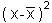628.755625 48.372025 0.133225 13.213225 241.958025 763.693225 0.112225 54.243225 1696.204225 876.456025

The average is (1,645.56+1,663.68+1,671.00+1,667.00+1,655.08+1,643.00+1,670.97+1,678.00+1,711.82+1,700.24) ÷ 10, which equals 1670.635. This figure of 1670.635 is our xbar (that is to say, our) value. For each of our closing prices, these being our x values, we deduct thevalue to give (x-), which is shown on the third row of the table above. Each (x-) value is then squared (ie multiplied by itself) to find thevalues in the fourth column. We then need to add all of thesevalues together to give the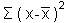value. In our case, thisis 628.755625 + 48.372025 + 0.133225 + 13.213225 + 241.958025 + 763.693225 + 0.112225 + 54.243225 + 1696.204225 + 876.456025, which equals 1354.52175.

We're nearly there - most of the complex calculations are now done. All we need to do now is the simple stuff. We know that, in our example, the value of n is 10 (this being the number of values in our data sample). So n-1 is 9. We need to divideby n-1, so ourvalue of 1354.52175 divided by 9 equals 150.502416667. This is our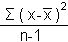value, so all we need to do now is to calculate the square root. For our example, the square root of 150.502416667 is 12.267943, so 12.267943 is our standard deviation value calculated in full.

More Technical analysis articles by Pandacash:

Plus more useful stocks and shares information:

Please bookmark Pandacash's Calculating Standard Deviation - Formula for Calculation in Stocks and Shares page for your future reference